Courses

# Prediction And Feasibility Of Spontaneity Of A Cell Reaction - Electro Chemistry Chemistry Notes | EduRev

## Chemistry : Prediction And Feasibility Of Spontaneity Of A Cell Reaction - Electro Chemistry Chemistry Notes | EduRev

The document Prediction And Feasibility Of Spontaneity Of A Cell Reaction - Electro Chemistry Chemistry Notes | EduRev is a part of the Chemistry Course Physical Chemistry.
All you need of Chemistry at this link: Chemistry

PREDICTION AND FEASIBILITY OF SPONTANIETY OF A CELL REACTION

Work done by the cell = nFE;

It is equivalent to decrease in free energy ΔG = -nFE
Under standard state ΔG0 = -nFE0 (i)

(i) From thermodynamics we know,ΔG = negative for spontaneous process. Thus from eq.(i) it is clear that the EMF should be +ve for a cell process to be feasible or spontaneous.
(ii) When   ΔG = positive, E = negative and the cell process will be non spontaneous.
(iii) When  G = 0 , E = 0 and the cell will attain the equilibrium.

Reactions                  ΔG        E
Spontaneous             (-)        (+)
Non- spontaneous    (+)        (-)
Equilibrium                0          0

Standard free energy change of a cell may be calculated by electrode potential data.
Substituting the value of  E0 (i.e., standard reduction potential of cathode- standard reduction potential of anode) in eq. (i) we may get ΔG0.
Let us see whether the cell (Daniell) is feasible or not: i.e. whether Zinc will displace copper or not.

Zn | (s) | ZnSO4 (sol) || CuSO4 (sol) | Cu(s)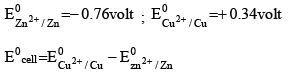=0.34 –(–0.76) = +1.10 volt
Since E0 = +ve , hence the cell will be feasible and zinc will displace copper from its salt solution. In the other words zinc will reduce copper.

THERMODYNAMIC TREATMENT OF NERNST EQUATION

Determination of equilibrium constant : We know, that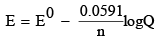..(i)

At equilibrium, the cell potential is zero because cell reactions are balanced, i.e. E = 0
∴ From Eq. (i), we have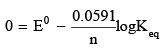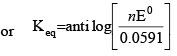Heat of Reaction inside the cell: Let n Faraday charge flows out of a cell of e.m.f. E, then -ΔG = nFE (i) Gibbs Helmholtz equation (from thermodynamics ) may be given as,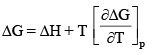.....(ii)
From Eqs. (i) and (ii), we have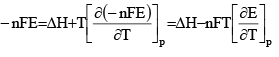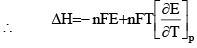Entropy change inside the cell : We know that G = H - TS      or ΔG = ΔH - TΔS .           ..(i)
where ΔG = Free energy change ; ΔH = Enthalpy change and ΔS = entropy change.
According to Gibbs Helmoholtz equation,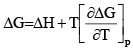....(ii)
From Eqs. (i) and (ii), we have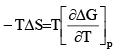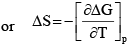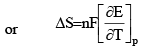where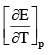is called temperature coefficient of cell e.m.f.

DIFFERENTTYPES OF HALF-CELLS  AND THEIR REDUCTION POTENTIAL

Gas-Ion Half: Cell In such a half cell, an inert collector of electrons, platinum or graphite is in contact with gas and a solution containing a specified ion. One of the most important gas-ion half cell is the hydrogen-gashydrogen ion half cell. In this half cell, purified H2 gas at a constant pressure is passed over a platinum electrode which is in contact with an acid solution.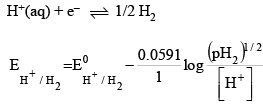Hydrogen gas Hydrgen ion Half cell

One of the most important gas-ion half-cell is the hydrogen gas-hydrogen in half-cell (also comonly known as hydrogen electrode). In this half-cell, purified hydrogen gas at a constant pressure is passed over a platinum electrode which is in contact with an acid solution.

Expression of Reduction Potential (Nernst Equation)

The expressionof Ehalf-cell can be derived by considering the reversible reduction reaction that occurs at the electrode. For the present case, we have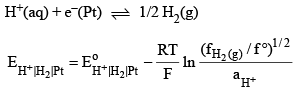Equation of the above type is known as the Nernst equation.

METAL-METAL ION HALF-CELL

Metal-metal ionhalf-cell consists of a bar of metal M in contact with a solution containing Mn+ ions.
Examples include zinc-zinc ion, copper-cupric ion, silver-silver ion and gold-auric ion half-cells.

Expression of Reduction Potential

The equilibrium reaction att he electrode is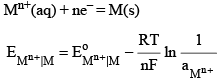METAL  AMALGAM-METAL ION HALF CELL

In this electrode, metal amalgam is placed in contact with a solution containing metal ion. Electrical contact is made by a platinum wire dipping into the amalgam pool.

Expression of Reduction Potential

The equilibrium reaction at the electrode is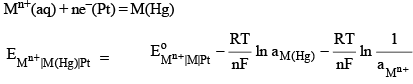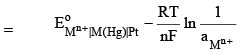where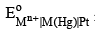is the standard potential of the given metal amalgam. Its value may be determined by using a solution of known activity of Mn+.

METAL-INSOLUBLE SALT-ANION HALF-CELL

In this half-cell, a metal coated with its insoluble salt is in contact with a solution containing the anion of the insoluble salt. Three such half-cell are described below.

Silver-Silver chloride chloride half-cell

This half cell is represented as Cl– | AgCl | Ag. The equilibrium reaction that occurs at the electrode is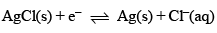Expression of Reduction Potential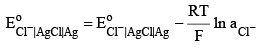Mercury-mercuric oxide-hydroxide Ion half-cell

In this half-cell, a pool mercury is covered with a paste of solid HgO and a solution of a base.  This equilibrium reaction that takes place at the platinum electrode is

HgO(s) + H2O(1) + 2e = Hg(1) + 2OH–(aq)

Expression of Reduction Potential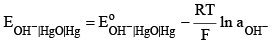Mercury-mercurous chloride-chloride ion half cell

This half-cell is known as calomel half-cell. The equilibrium reaction is
Hg2Cl2(s) + 2e = 2Hg(l) + 2HCl(aq)

Its Nernst equation is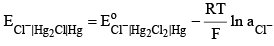OXIDATION REDUCTION HALF-CELL

An oxidation reduction half-cell has an inert metal collector, usually platinum, immersed in a solution which contains two ions of the same elements in different states of oxidation.

Ferric-ferrous half-cell
Fe3+(aq) + e-(Pt) = Fe2+(aq)

The Nernst equation is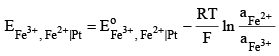Stannic-Stannous Half-cell

Another example is stannic-stannous half-cell where the reduction reaction to be considered is

Sn4+(aq) + 2e = Sn2+(aq)
and the Nernst equation is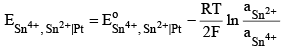Reference half-cell :

If EL is arbitrarily assigned some vlaue, then the value of ER can be determined using the expression
ER = Ecell + EL
In the study of electrochemical cell, the hydrogen-hydrogen ion half-cell has been adopted as the reference half-cell and its standard potential has been assigned the vlaue zero at all temperatures.

By standard potential of hydrogen-hydrogen ion half-cell, we mean that the hydrogen ion and hydrogen gas involved in half-cell.
H+(aq) | H2(g) | Pt
are present in their standard states of unit activity and unit fugacity (taken as 1 bar pressure), respectively.
ER = Ecell + 0 = Ecell

Illustrations

To illustrate the procedure, we cite below two typical examples of silver-silver ion and zinc-zinc ion half-cells. If the silver-silver ion half-cell is coupled with the standard hydrogen-hydrogen ion half-cell (to be kept on left side), we get a cell

Pt | H2(1 bar) | H+(a = 1) || Ag+(a = 1) | Ag

The emf of the cell is given by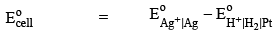and its value as determined experimentally is found to be 0.799 1 V.  Hence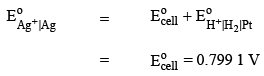Taking the example of zinc-zinc ion half-cell, we have

Pt | H2(1 bar) | H+(a = 1) || Zn2+(a = 1) | Zn

Its emf as determined experimentally is found to be – 0.763 V. Hence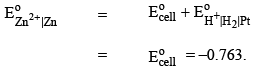INFLUENCE OF IONIC ACTIVITY ON REDUCTION POTENTIAL

The reduction potential for the reaction

Mn+ + ne = M
as given by the Nernst equation is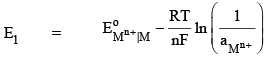It follows that the reduction potential EoMn+|M depends on the activty of Mn+; it increases as the activity of Mn+ is decreased to one-tenth of its original activity, then the reduction potential becomes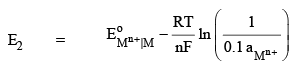The resulting change in potential is given by

E2 – E1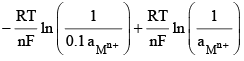=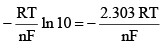At 298 K, we have

E– E1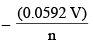Thus every ten-fold decrease in the activity of cation produces a decrease of (0.059 2/n) volt of the reduction potential, where n is the valence of the cation. For univalent cations n is 1, and hence reduction potential decreases by a factor of 0.059 2 V. For bivalent cations n is 2, and hence reduction potential decreases by a factor of (0.059 2/2) V, i.e. 0.029 6 V. The magnitude of reduction potential will change by the same factor (= 0.0592/n volt) for every ten-fold change in the activity of cation. For a hunderedfold change in the activity; the change of potential will be 2 × 0.059 2/n volt and for a thousand-fold change in activity, the potential will change by a factor of 3 × 0.059 2/n volt.

Relation between metal-metal ion half-cell and the corresponding metal-insoluble salt-anion half-cell

Consider a silver electrode dipped into a solution of silver nitrate. The potential of the electrode as given by the Nernst equation is
E0Ag+|Ag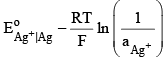with EoAg+|Ag = 0.799 V

If now NaCl is added, the following equilibrium will be established :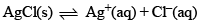with its solubility product Ksp as

Ksp = (aAg+ ) (aCl- )

Multiplying and dividing the term within parantheses in eq. (i) by aCl- , we get

E0Ag+|Ag =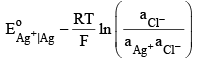E0Ag+|Ag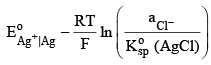E0Ag+|Ag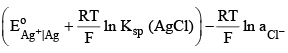Now the electrode can also be treated as Ag | Agcl | Cl since part of AgCl will deposit on solid Ag.
Writing the equilibrium expression for this electrode, we have

AgCl(s) + e = Ag(s) + Cl(aq)

The corresponding Nernst equation gives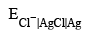=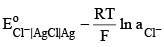Above equation must be indentical and hence on comparing these two equations, we get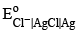=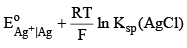or=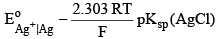where pKsp(AgCl) = – log Ksp(AgCl)

Cell reaction and its relation with cell potential

ΔG = -nF(ER - EL) = - nFEcell

we consdier the various ways in which the following three half-cell reactions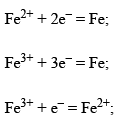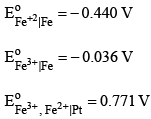may be combined to form a cell with the following overall cell reaction.

Fe + 2Fe3+ = 3Fe2+.

Combination of Equations:

2Fe3+ + 6e = 2Fe; EoFe3+ |Fe = - 0.036 V

3Fe2+ + 6e– = 3Fe; EoFe+2|Fe = - 0.440 V
with the overall reaction
Fe3+ + 3Fe3+ = 3Fe2+; Eocell = 0.404 V
The cell producting this reaction would be
Fe | Fe2+ || Fe3+ | Fe

REFERENCE HALF CELLS

Common examples of reference half-cell
The most common type of reference half-cell has the following form :Examples include :

(1)  Calomel half-cell : KCl(aq) | Hg2Cl2(s) | Hg

(2)  Silver-silver chloride half-cell : KCl(aq) | AgCl(s) | Ag

(3)  Mercury-mercurous sulphate half-cell : K2SO4(aq) | Hg2SO4(s) | Hg

Calomel half-cell :

The half-cell reaction is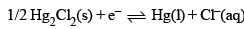and the Nernst equation has the form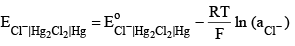with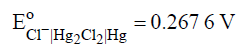Thus, the calomel half-cell functions as the reversible chloride electrode.

Silver-Silver chloride Half-cell

The half-cell reaction is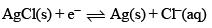Hence the half cell potential is given by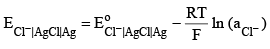with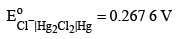This half-cell cannot be used in a solution which contains HNO3, I and CN ions.

Mercury-Mercurous sulphate half-cell
The half-cell set up in very similar to that of calomel half-cell. the cell-reaction is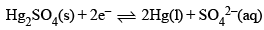Hence the half-cell potential is given by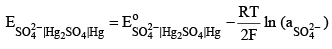with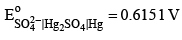This half-cell potential depends upon the activity of SO42- ions.

Mercury-mercuric oxide half-cell
The half-cell reaction is
1/2 HgO(s) + 1/2H2O + e = 1/2 Hg(l) + OH(aq)
and thus the Nernst equation has the form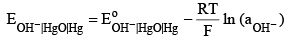Offer running on EduRev: Apply code STAYHOME200 to get INR 200 off on our premium plan EduRev Infinity!

## Physical Chemistry

66 videos|94 docs|32 tests

,

,

,

,

,

,

,

,

,

,

,

,

,

,

,

,

,

,

,

,

,

;